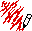## The Problem

Here's the definition of `intersect` given by Graham in Chapter 9, extended to show what it would like if cubes were added:

```(defun intersect (s pt xr yr zr)
(funcall (typecase s
(sphere #'sphere-intersect)
(cube #'cube-intersect))
s pt xr yr zr))```

As noted, this approach means that we will have to redefine `intersect` every time we define a new kind of object . Furthermore, whereever `intersect` should go, it definitely doesn't belong in the spheres definition file, because of the reference to cubes. On the other hand, we'd rather not put it in the base file where `tracer` and so on are defined because

• it would make the base file dependent on every object definition file
• we'd have to edit the base file and recompile it every time we added a new kind of object
• we'll get "undefined function" errors when `intersect` is compiled if any of the object files are missing, even if we don't need them

## The Object-Oriented Solution

The minimal change to make Graham's code object-oriented is to define intersect in the base file as a method on surfaces:

```(defmethod intersect ((s surface) pt xr yr zr)
(error "Unknown surface ~S" s))```

This is the default method. It signals an error because there is no default way to do ray intersection on an unknown kind of surface.

In each object definition file, we'd define the appropriate intersection method for the object, e.g.,

```(defmethod intersect ((s sphere) pt xr yr zr)
(let* ((c (sphere-center s))
(n ...))
...)```

That's all we need to do. When Common Lisp sees a call of the form `(intersect ``object``)`, it will determine the appropriate method to use, based on the object.

Our base file makes no explicit reference to any kind of object, and each object file is independent of any other object file.

Note: it would be more typical object-oriented programming to replace the structures with classes. In the base file, we put:

```(defclass surface ()
((color :accessor surface-color :initarg :color)))```

In the sphere definition file, we'd put:

```(defclass sphere (surface)
Comments?Send mail to Chris Riesbeck.